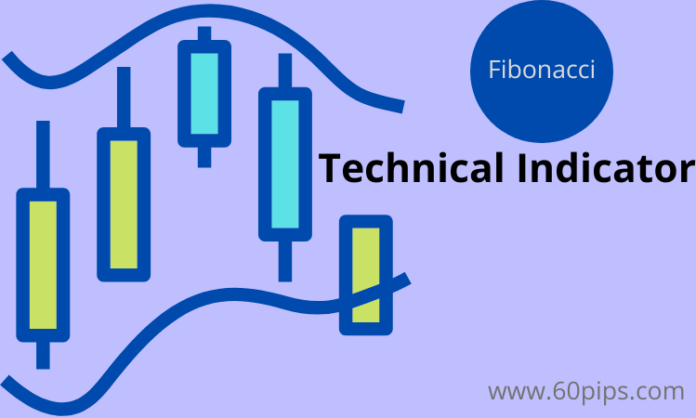# Fibonacci retracement in Forex mt4 Trading platform

22A Fibonacci retracement is a technical analysis tool used to find potential reversal points in the market by measuring the length of a price trend and then dividing that length by Fibonacci ratios.

The most common Fibonacci ratios used are 0.382, 0.5, and 0.618 which are derived from the mathematical sequence discovered by Leonardo Pisano Bigollo, also known as Fibonacci.

Fibonacci retracements can be applied to any time frame but are most commonly used on daily and weekly charts. The tool is made up of five horizontal lines which represent different levels of support or resistance: 23.6%, 38.2%, 50%, 61.8% and 100%.

When prices reach one of these levels, it is often seen as an opportunity for traders to enter into a trade or exit their current position. There are other important technical Indicators are RSI, MA and awesome oscilator.